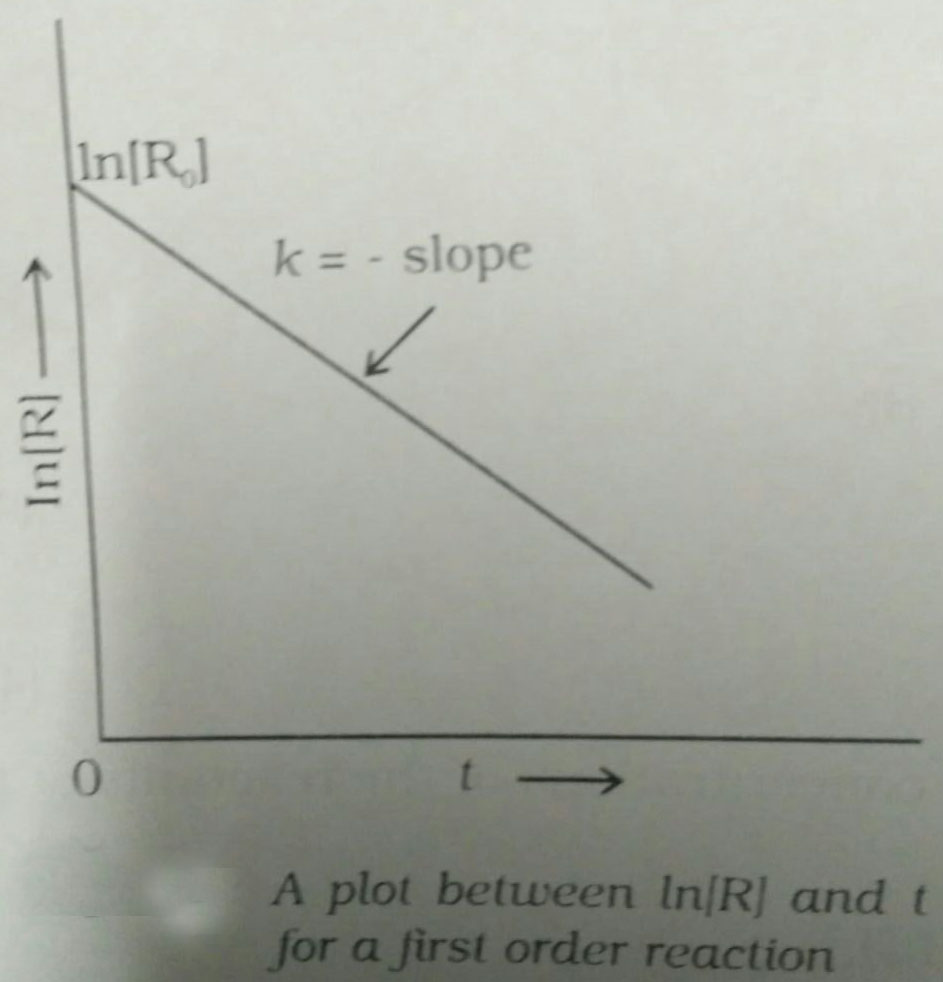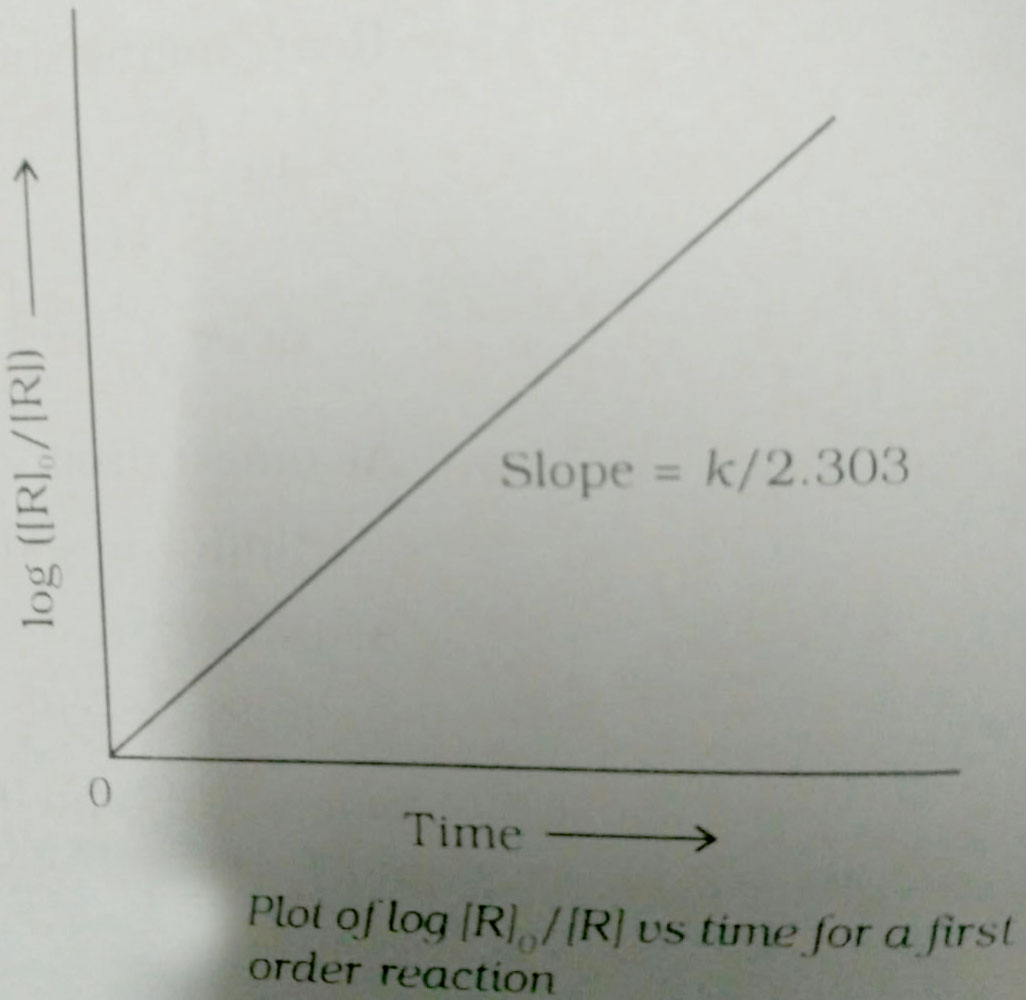Q

# Help me solve this If half-­life of a substance is 5 yrs, then the total amount of substance left after 15 years, when initial amount is 64 grams is

If half-­life of a substance is 5 yrs, then the total amount of substance left after 15 years, when initial amount is 64 grams is

• Option 1)

16 grams

• Option 2)

2 grams

• Option 3)

32 grams

• Option 4)

8 grams

197 Views

As learnt in concept

First Order Reaction -

The rate of the reaction is proportional to the first power of the concentration of the reaction- wherein

Formula:

R            P

a                 0

a-x             x

[differentiate rate law]

Unit of

T1/2 = 5 years

X = 8 gm

Option 1)

16 grams

This option is incorrect.

Option 2)

2 grams

This option is incorrect.

Option 3)

32 grams

This option is incorrect.

Option 4)

8 grams

This option is correct.

Exams
Articles
Questions